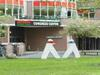# Cancelled: 4th QSC General Assembly

This General Assembly has been cancelled, because of the corona virus.

Chair

• Harry Buhrman – Center for Mathematics and Computer Science & University of Amsterdam
• Jop Briët – Center for Mathematics and Computer ScienceThe track Quantum Networks is jointly organised with the Gravitation programme Networks.

This track is about network phenomena in quantum information science, a field whose main aim is to explore computational and information-theoretic aspects of quantum mechanics. Key examples include quantum algorithms for classical problems, for instance to study large graphs, networks of quantum systems, where strange interactions connect nodes of separated quantum systems, communication networks featuring quantum-enhanced channels or channels for transmitting quantum information itself, and computational complexity theory under the presence of quantum devices and agents.
These topics enjoy lively interactions with a broad range of mathematical areas, including combinatorics, functional analysis, algebra and probability. Some of the state of the art on these themes will be presented by world experts.

### Speakers

#### Debbie Leung University of Waterloo/Perimeter Institute for Theoretical PhysicsCapacity Approaching Coding for Low Noise Interactive Quantum Communication

Abstract: We consider an arbitrary two-party interactive quantum communication protocol P of n noiseless quantum messages.  We provide an interactive protocol P’ using n(1+\Theta(\sqrt(e)) messages of which a fraction e can be corrupted adversarially.  The protocol P’ simulates P with failure probability vanishing exponentially in ne.

In this talk, we will describe the problem in detail, why traditional quantum error correcting codes cannot be used, summarize Haeupler’s solution for the analogous classical problem, and several quantum twists required to adapt Haeupler’s solution to the quantum setting.

Joint work with Nayak, Shayeghi, Touchette, Yao, and Yu.

#### Frédéric Magniez CNRS, IRIF, Université de ParisQuantum algorithms for graphs and networks

Abstract: We will overview several quantum paradigms including quantum amplification, quantum walks and quantum sampling, and some of their applications for solving graph problems, estimating statistics, and designing distributed algorithms. While giving insights on the quantum tools used in those paradigms, we will provide simple frameworks for designing non-quantum algorithms that can be automatically and quantumly sped up, without any prior knowledge on quantum computing. We will demonstrate our methodology by presenting several algorithms for basic but yet fundamental problems such as triangle detection and counting, and diameter computation in a network.

#### Laura Mancinska QMATH, University of CopenhagenQuantum isomorphism is equivalent to equality of homomorphism counts from planar graphs

Abstract: Over 50 years ago, Lovász proved that two graphs are isomorphic if and only if they admit the same number of homomorphisms from any graph [Acta Math. Hungar. 18 (1967), pp. 321–328]. In this talk we will see that two graphs are quantum isomorphic if and only if they admit the same number of homomorphisms from any planar graph.

#### Henry YuenThe complexity of games with entanglement

Abstract: In this talk, I will discuss recent advances in understanding the complexity of games involving entangled players. Remarkably, these complexity-theoretic results have implications for foundational questions in quantum information theory, as well as in pure mathematics. I will give an overview of the connections between quantum entanglement, the computable, and the uncomputable.

https://www.networks2020.nl/scientific-conference/quantum-networks/

DetailsWhen: Friday June 12th, 2020
Time: 09:30 - 17:30
Where: Amsterdam Science Park, CWI

Science Park 123, 1098 XG Amsterdam